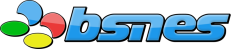# CPU | ALU

These are algorithms to compute the overflow, carry, and half-carry (Z80 family) flags for addition and subtraction operations, without the use of branches.

They are designed to not require extra bits in the result computation, so that they can be templated to larger-sized types, and be used even with the largest type a given machine is capable of using.

Of course, to template these, you will need a portable way to extract the highest bit of a given integer type for the overflow and carry masks, eg:

```				```
natural sign = (natural(0) - 1 >> 1) + 1;
```
```

In the below examples, natural can be any type from uint1 to uintmax. boolean has the same behavior as bool: output = input ? true : false — that is to say, value & bit will subtract a desired bit, rather than value >> bit – 1 & 1.

```				```
auto adc(natural target, natural source, boolean carry) -> uint8 {
natural result   = target + source + carry;
natural carries  = target ^ source ^ result;
natural overflow = (target ^ result) & (source ^ result);

flag.overflow  = overflow & sign;
flag.carry     = (carries ^ overflow) & sign;
flag.halfCarry = carries & 16;  //Z80

return result;
}
```
```

## SBB: subtract with borrow

Nearly all processors implementation subtraction via borrow. Certain CPU vendors have confused this, and named their implementations SBC (subtract with carry), even though that is a different operation. A notable example here is the Toshiba TLCS900H.

```				```
auto sbb(natural target, natural source, boolean carry) -> uint8 {
natural result   = target - source - carry;
natural carries  = target ^ source ^ result;
natural overflow = (target ^ result) & (source ^ target);

flag.overflow  = overflow & sign;
flag.carry     = (carries ^ overflow) & sign;
flag.halfCarry = carries & 16;  //Z80

return result;
}
```
```

```				```
auto sbb(natural target, natural source, boolean carry) -> uint8 {
natural result = adc(target, ~source, !carry);

flag.carry     = !flag.carry;
flag.halfCarry = !flag.halfCarry;

return result;
}
```
```

## SBC: subtract with carry

The 6502 series of processors (MOS6502, Ricoh 6502, HuC6280, WDC65816) use carry rather than borrow for subtraction.
```				```
auto sbc(natural target, natural source, boolean carry) -> uint8 {
natural result   = target - source - !carry;
natural carries  = target ^ ~source ^ result;
natural overflow = (target ^ result) & (source ^ target);

flag.overflow  = overflow & sign;
flag.carry     = (carries ^ overflow) & sign;
flag.halfCarry = carries & 16;  //Z80

return result;
}
```
```

The reason for this becomes clear when looking at an alternate implementation:
```				```
auto sbc(natural target, natural source, boolean carry) -> uint8 {
}
```
```
With the 6502 weighing in at just 3500 transistors, it becomes advantageous to be able to reuse the ADC circuitry for SBC. Although this can be done with SBB as well, it is more complicated due to the flags needing to be inverted.

## Thanks

Special thanks to Talarubi for the insight into creating a branchless carry computation!